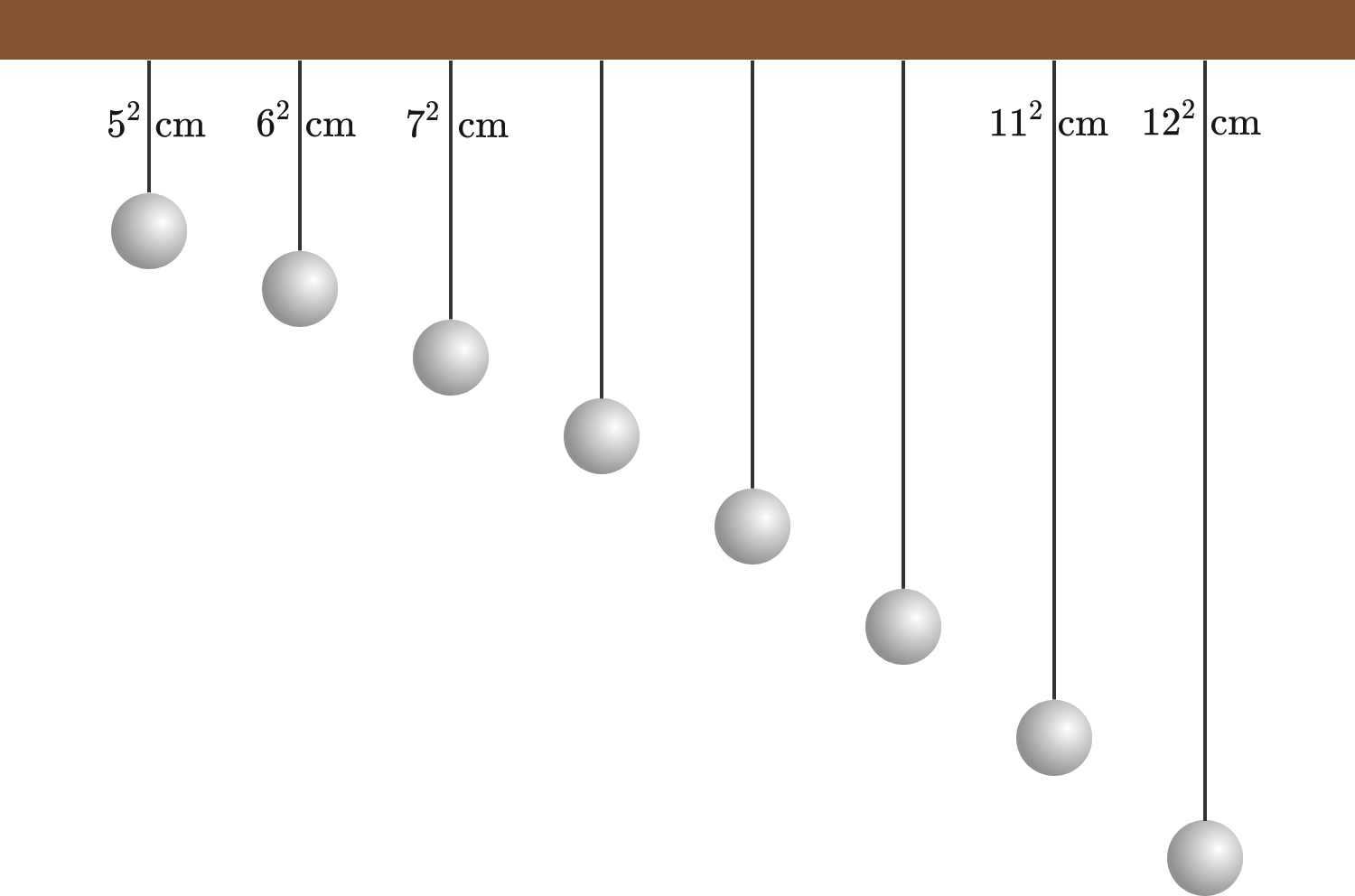# Pendulums, pendulums everywhere

A simple pendulum consists of a small object dangling from a piece of string. A creative student constructs 8 pendulums of different lengths: $5^2 \text{ cm},\ 6^2 \text{ cm},\ 7^2 \text{ cm},\ ...,\ 11^2 \text{ cm},\ 12^2 \text{ cm},$ and hangs them next to each other in increasing order as shown in the diagram below. Thus, $L_n = \SI{(n+4)^2}{cm}$: the shortest pendulum has length $L_1 = \SI{25}{cm},$ and the longest $L_8 = \SI{144}{cm}$.

The student now starts the pendulums, by pulling them all sideways over the same short distance and releasing them at the same time $t = 0$. Because of their different lengths, the pendulums swing at different frequencies and will soon all be completely out of phase.

How long will it take for all these pendulums to be exactly in phase again?Details and Assumptions:

• $g = \SI{9.81}{m/s^2}.$
• The pendulums are ideal, simple pendulums with period $T_n = 2\pi\sqrt{\frac {L_n}g}.$
• For a demonstration of this kind of setup, see this video.
×

Problem Loading...

Note Loading...

Set Loading...